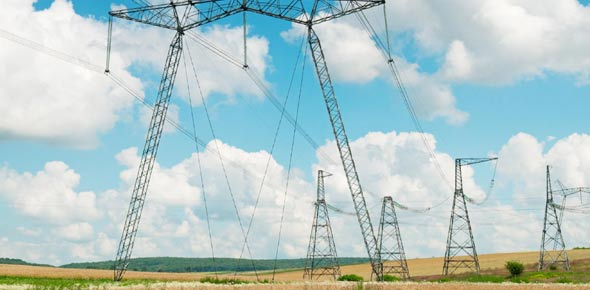# MCQ Quiz: Electricity Test! Trivia

23 Questions | Total Attempts: 402SettingsMCQ quiz: electricity test trivia. Electricity is can either be static, current, hydro or solar. It is used for heating and lighting, and to provide power for machines. Electricity can either be sourced from friction, chemical action, light, heat, pressure, and magnetism. How do you chance electric energy from one form to another? Refresh your memory by taking this quiz and see what more you will learn about electricity.

Related Topics
• 1.
Circuit with more than one path.
• A.

Parallel

• B.

Series

• 2.
A material that current does not easily flow through:
• A.

Conductor

• B.

Insulator

• C.

Electric discharge

• D.

Voltage

• 3.
The rapid movement of excess electrons from one place to another.
• A.

Static electricity

• B.

Insulator

• C.

Electric discharge

• D.

Series

• 4.
The rate at which electric energy is converted to another form.
• A.

Electric field

• B.

Static charge

• C.

Circuit

• D.

Power

• 5.
The closed path through which a current can flow.
• A.

Circuit

• B.

Voltage

• C.

Resistance

• D.

Conductor

• 6.
The buildup of electrical charges in one place.
• A.

Current electricity

• B.

Ohm's Law

• C.

Static charge

• D.

Electrical power

• 7.
Exists around every electric charge.
• A.

Insulator

• B.

Electric field

• C.

Voltage

• D.

Electric discharge

• 8.
• A.

Static charge

• B.

Current

• C.

Electric field

• D.

Voltage

• 9.
The measure of how difficult it is for electrons to flow.
• A.

Series

• B.

Resistance

• C.

Conductor

• D.

Static charge

• 10.
A material that current flows through easily.
• A.

Conductor

• B.

Insulator

• C.

Electric discharge

• D.

Parallel

• 11.
An atom with a positive or negative charge.
• A.

Electric field

• B.

Resistance

• C.

Parallel circuit

• D.

Ion

• 12.
A circuit with more than one path along which current can flow
• A.

Series

• B.

Parallel

• C.

Electric discharge

• D.

Resistance

• 13.
Place a checkmark beside all the following that are good conductors.
• A.

Dry skin

• B.

Water

• C.

Rubber

• D.

Iron

• E.

Copper

• F.

Styrofoam

• 14.
Place a checkmark beside all of the insulators.
• A.

Iron

• B.

Rubber

• C.

Dry skin

• D.

Copper

• E.

Styrofoam

• F.

Water

• 15.
A toaster is plugged into a 110-volt outlet.  What is the resistance of the toaster if the current in the toaster is 10 A?  Write your answer to the nearest 10th.  _____Ohms.
• 16.
A hair dryer uses 1,000 W when it is plugged into a 110-volt outlet.  What is the current in the hair dryer?  Write your answer to the nearest 10th.  ______A.
• 17.
A lightbulb with a resistance of 10 ohms is connected to a battery.  If the current in the lightbulb is 0.10 A, what is the voltage of the battery?  Write your answer to the nearest 10th.  ____ V.
• 18.
Which of these things does a circuit need to work properly?
• A.

Bulb

• B.

A power supply

• C.

Crocodile clips

• D.

Plastic

• 19.
If a circuit is not complete
• A.

It will not work

• B.

It might work

• C.

It will work

• D.

The bulbs glow

• 20.
What do cells (batteries) do in a circuit?
• A.

Nothing

• B.

Light up

• C.

Provide electricity

• D.

Use up electricity

• 21.
Will the following series circuit work?
• A.

Yes, it is a complete circuit.

• B.

No, it is not a complete circuit.

• 22.
Will the following LED light light up?
• A.

Yes, it is a complete circuit

• B.

No, the switches are open

• C.

No, the switches are closed

• 23.
Why do circuits need to be complete?
• A.

To make them round in shape

• B.

So the wires are all the same size

• C.

To make the bulbs go out

• D.

So that the electricity can travel all the way around